Documentation

This is machine translation

Mouseover text to see original. Click the button below to return to the English version of the page.

Improving Stability While Preserving Open-Loop Characteristics

This example shows how to use Robust Control Toolbox™ function `ncfsyn` to improve the stability robustness of a closed-loop system while approximately maintaining the high-gain and low-gain characteristics of the open-loop response.

Plant Model

The plant model is a lightly-damped, second-order system

``

A Bode plot clearly shows the resonant peak.

```P = tf(16,[1 0.16 16]); bode(P) ```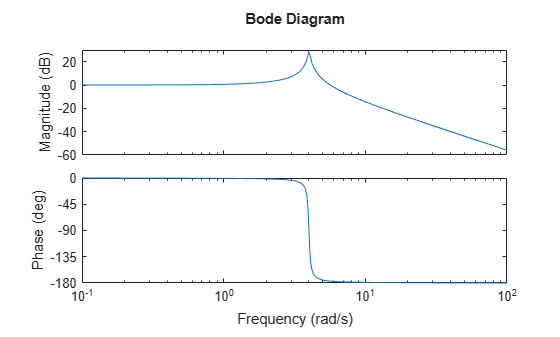Closed-Loop Objectives and Initial Controller Design

The closed-loop system should

• Have integral action, and a sensitivity bandwidth of at least 0.5 rad/sec

• Have gain-crossover frequencies no larger than 7

We can translate these requirements into a desired shape for the open-loop gain and seek a controller that enforces this shape. For example, a controller `Kprop` consisting of a PI term in series with a high-frequency lag component achieves the desired loop shape:

```K_PI = pid(1,0.8); K_rolloff = tf(1,[1/20 1]); Kprop = K_PI*K_rolloff; bodemag(P*Kprop); grid ```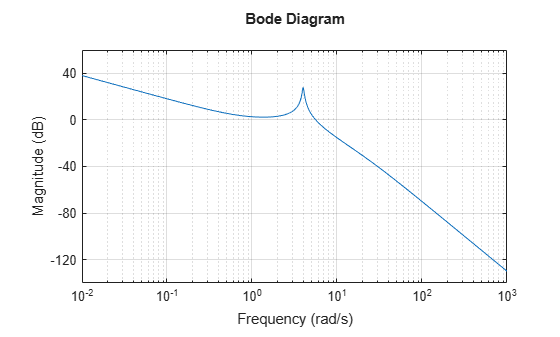The desired high-frequency rolloff, integral action, and location of the gain-crossover frequency are all evident from the graph. Unfortunately, this controller does not stabilize the closed-loop system, as evidenced by the `Stable` field from the `allmargin` calculation.

```allmargin(P*Kprop) ```
```ans = struct with fields: GainMargin: 0.1096 GMFrequency: 4.1967 PhaseMargin: -20.4811 PMFrequency: 5.6155 DelayMargin: 1.0552 DMFrequency: 5.6155 Stable: 0 ```

Closed-loop Stability and Margin Improvement Using `ncfsyn`

The command `ncfsyn` can be used to obtain closed-loop stability and improved stability margins without significant degradation in the large (>>1) and small (<<1) gain regions of the open-loop response. A value of `Gamma` (the 3rd output argument) less than 3 indicates success (modest gain degradation along with acceptable robustness margins). Note that `ncfsyn` assumes positive feedback so you must flip the sign of `Kprop`. The new controller, K, stabilizes the nominal dynamics, and appears to have decent robustness margins, at least when measured in the classical sense.

```[negK,~,Gamma] = ncfsyn(P,-Kprop); K = -negK; % flip sign back Gamma allmargin(P*K) ```
```Gamma = 1.9883 ans = struct with fields: GainMargin: [6.3299 11.1423] GMFrequency: [1.6110 15.1667] PhaseMargin: [80.0276 -99.6641 63.7989] PMFrequency: [0.4472 3.1460 5.2319] DelayMargin: [3.1236 1.4443 0.2128] DMFrequency: [0.4472 3.1460 5.2319] Stable: 1 ```

As expected, the closed-loop is stable, and with `Gamma` less than 2, the gain and phase margins are adequate by rule-of-thumb standards.

Bode Magnitude of Controllers

`Gamma` gives an indication of the gain degradation (in going from `Kprop` to `K`) we should expect, both the in the large and small loop-gain regions. With `Gamma` approximately 2, we expect only about 6dB (20*log10(Gamma)) gain reduction in the large loop-gain regions, and no more than 6dB gain increase in the small loop-gain regions. Bode magnitude plots confirm this.

```subplot(1,2,1) bodemag(Kprop,'r',K,'g'); grid legend('Proposed Controller, Kprop','Redesigned Controller, K') title('Controller Gains') subplot(1,2,2) bodemag(P*Kprop,'r',P*K,'g'); grid legend('P*Kprop','P*K') title('Open-Loop Gains') ```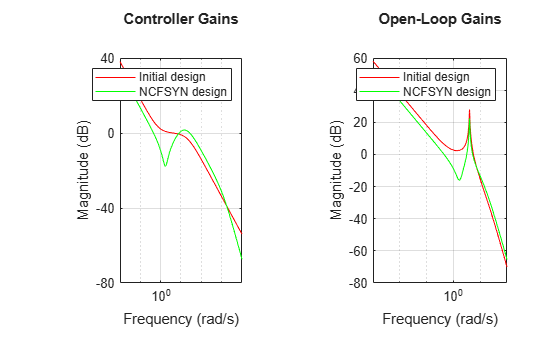Figure 1: Controller and Open-Loop gains

Closed-Loop Response to Impulse at the Plant Input

An impulsive disturbance at the plant input is damped out effectively in a few seconds. For comparison, the uncompensated plant response is also shown.

```TF = 4; subplot(1,2,1) impulse(feedback(P,K),'b',P,'r',TF); legend('Closed-Loop','Open-Loop') title('Plant response, y, to impulse at plant input') subplot(1,2,2); impulse(-feedback(K*P,1),'b',TF) legend('Closed-Loop Control action, u') ```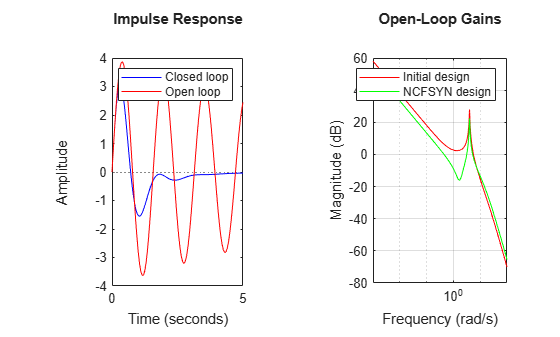Figure 2: Controller and Open-Loop gains

Sensitivity and Complementary Sensitivity Functions

Finally, the closed-loop Sensitivity and Complementary Sensitivity functions show the desired sensitivity reduction and high-frequency noise attenuation expressed in the closed-loop performance objectives.

```S = feedback(1,P*K); T = 1-S; clf bodemag(S,T,{1e-2,1e2}); grid ```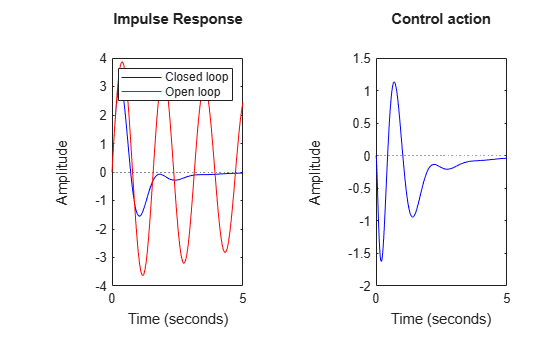Conclusions

In this example, the function `ncfsyn` is used to adjust a proposed controller in order to achieve closed-loop stability, while attempting to maintain the general large and small loop-gain characteristics of the open-loop function `PK`.

Robust Control Toolbox Documentation평가판 신청